## Open Economy: the Disequilibrium Macromodel of Exchange Rates and Its Stability

Wang Qifan Chen Zhanqiang Zhou Minghai

School of Management,Fudan University,Shanghai,200433,China

Economists have paid their great attentions to the macroeconomics study under open economy. In this paper, from viewpoints of Systems thinking, we considered such variables as aggregate supply of products, money supply and wage endogenous. Based on Dornbusch Model, we established the disequilibrium model including exchange rates,price of products,foreign assets and wage. Finally,we gave the stablity conditions for the model.

I. The History

With the rapid development of economy and science & technology ,the world economy is becoming more and more globalized,and the good way to develop the national economy is to expand the scale of its openess to the outside world.Accordingly, world economists have attached great importance to macroeconomics under open economy.

In macroeconomics under open economy, much original research work has been done by many economists such as Robert Mundell, Marcus Fleming and Rudiger Dornbusch in the1960s.The Mundell-Fleming Theory [1,2] enlarged the IS-LM model to open economy. The M-F model considered a small country economy where the monetary system is under floating exchange rates, the price is fixed, the capital movement is incomplete and the expectation of exchange rates is static. The emphasis of the model was on the different conditions which determined the balance of capital account and net inflow of capital. The M-F theory was very popular in the1960s, but the main defect of M-F is the assumption of the fixed price and static expectation of exchange rates which are impractical for a long-term economic activity. For this, Dornbusch,the world-famous economist in MIT put forward his famous Dornbusch Model  to revise M-F model in 1976.Based on M-F model, Prof. Dornbusch put into his model the adjustment of price of products,complete capital movement and complete expectation of exchange rates to make it more practical in real world.

2. The Problem in the models

The socio-economic system is a complicated system,where many variables are relative and interactive. Capable as Dornbusch model is in describing and explaining the real world , it merely explores the relationship between exchange rates and price, and regards many important variables such as aggregate supply of products, money supply as exogenous. In fact, these variables are heavily interactive with price and exchange rates. Based on Dornbusch model and from the viewpoints of Systems thinking, we made the following variables as endogenous. They are aggregate supply of products,money supply, and wage. Moreover, we establish the disequilibrium model including exchange rates, price, foreign assets and wage. Here, we consider the open economy situation in a small country where the monetary system is under floating exchange rates, the capital movement and expectation of exchange rates are complete. As a small country, its economic activities have little impact on the world economy, but the world

economy greatly affects the country.

3. The Model

This model described the case in which the aggregate supply curve is in the middle area(not in keynes area or classical area).

In money market,the real demand of money can be written as

(1) m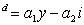where y is GNP,i is domestic interest rate.and the real demand of money equates to supply when the money market gets to an equilibrium,i.e.

(2)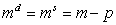where m is nominal supply of money. For the supply of basic money H,we have H=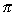F+D,where F is foreign asset in center bank, D is domestic credit(regarded as a constant here),is the nominal exchange rate. Setting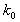as money multiplier,we can write the nominal supply of money as

(3) m=(F+D)

In product market,the aggregate demand of products is given by

(4)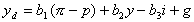where g is the government demand of purchase. In product market,the aggregate supply is determined by the function of production y=y(N,K), here we didnt consider the change of technology and capital, so the function of production is stable and the capital K is fixed.Here the supply of labour force N becomes the determining factor to products y, but the supply of labour force N is determined by the real wage. And finally, the aggregate demand of products is determined by the real wage. So we have

(5)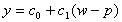where W is nominal wage.Setting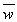as the long-term expected wage,the adjustment of nominal wage is

(6)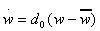We described the process of price adjustment as below

(7)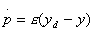where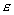is the speed of price adjustment.

The change of foreign assets should be the sum of net exporting value,interest of foreign assets and net inflow of capital. The capital account includes long-term capital and short-term capital. But the short-term capital has a great mobility,and has become the main factor which results in volatility of exchange rates in the international finance market. With the great progress of world economy and information technology, the amount of short-term capital investment is becoming larger and larger,and the mobility more complete.In our model,we just considered the net inflow of short-term capital,which is mainly determined by domestic market interest rate.Settingas foreign interest rate,we obtained

(8)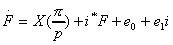where X() is net exporting value,is real exchange rate. Here we also took the assumptions that the capital movement and expectation of exchange rates are complete,

and by UIRP theory ,we had

(9)by (1),(2) and (3),we got

(10) i=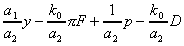substituting (10),(5) into (9),we got

(11)substituting (4),(5) and (10) into (7),we got

(12)substituting (5),(10) into (8),we got

(13)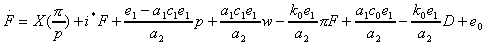For the sake of convenience, we denote the right of equation (11),(12),(13) and (6) as F1(,p,F,w),F2(,p,F,w),F3(,p,F,w) and F4(,p,F,w) respectively. From above,we obtained the Disequilibrium Macro-model including variables,p,F and w, i.e., the differential equations as below

(14)4. The Equilibrium Point and Stability of Model

Here we discussed a general form of system (14),and F1,F2,F3 ,F4 is a general function respectively.In autonomous system (14), given F1,F2,F3 ,F4 as zero,we reached the equilibrium point of system (14) (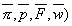. General speaking, the equilibrium points of system (14) are not unique , so here we just only considered the case when the equilibrium points of system are unique.

To discuss the stability of system (14) near the equilibrium point of system (14) (,we derivated the functions F1,F2,F3 ,F4 in system (14) with respect to variables,p,F,w,and obtained

A(,p,F,w)=Near the equilibrium point of system (14) (,if the eigenvalues of matix A(,p,F,w)(i=1,2,3,4), have negative real part, i.e., Re<0, the equilibrium point (is a stable point.

References

 Robert A.Mudell(1963)Capital mobility and Stabilization Policy under fixed and flexible exchnaeg rates",Canadian Journal of Economics and Political Science 78,475-485.

 Marcus Fleming(1962)Domestic financial under fixed and under floating exchange rates",IMF staff papers 3,369-380.

 Rudiger Dornbusch(1976)Expectations and exchange rate dynamics",Journal of Political Economy 84,1161-1176.

 Laurence S.Copeland(1989) Exchange Rate and International Finance, Addison-Wesley Publishing Company.

ISDC '97 CD Sponsor